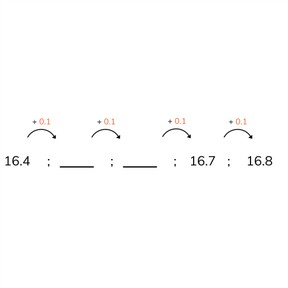Place value- Counting with decimal numbers with 1 or 2 decimal places

# Place value- Counting with decimal numbers with 1 or 2 decimal places

Place value- Counting with decimal numbers with 1 or 2 decimal places

No account needed.8,000 schools use Gynzy92,000 teachers use Gynzy1,600,000 students use Gynzy

## General

Students learn to count forwards and backwards with decimal numbers with 1 or 2 decimal places.

4.NF.C.5
4.NF.C.6

## Relevance

Students need to be able to do this to calculate using decimal numbers.

## Introduction

Start by naming the decimal numbers using degrees Fahrenheit and money amounts. Drag the correct temperature to the correct image. Ask students to say the numbers out loud. You can also ask them which is the highest and lowest number.

## Development

Discuss the distinction between a decimal number, a decimal point and decimal place with your students. Decimal place(s) is/are the numbers that come after the decimal point, and you can have many numbers after the decimal point! Using the number line, show that you can make ten jumps of 0.1 to go from 0 to 1. Using a battery show what 0.1 represents. Every line is 10% and when it is completely full it is 100%. Using another similar exercise and the number line, check that students understand counting on in tenths. Next repeat the instruction, but counting in steps of 0.01. Use the visual of 1 cent (\$0.01). Spend time at the end explaining the steps from 9 to the next tenth or hundredth, for example from 5.9 to 6.0 or 6.99 to 7.00.

Check that students understand counting forward and backwards with up to 2 decimal places by asking the following questions:
- How can you tell if you are counting in steps of 0.1 or 0.01? (look at the start and end numbers of the number line)
- When do you count on to the next number? (from 0.9 or 0.99)

## Guided practice

Students first complete a number sequence by counting on (including counting through to a next tenth or hundredth). They first count by 0.1, then by 0.5. The first exercise asks students to "Write all the numbers with one decimal place" indicating that they should write, for example, 6.0.

## Closing

Emphasize that it is important to be able to count forwards and backwards with decimal numbers so that they can calculate using decimal numbers in the future. Discuss decimal numbers with your students. When do you see them in daily life? Examples include temperature, in stores, weight, using timers, etc. Finish with a game. As a class, you'll make a "snake" of decimal numbers. Stand in a line and give the first student a number, say 4.0. They next student must name the number +0.1. That means the snake continues with 4.1, 4.2, 4.3, etc. You can repeat this a few times before starting with a new head for the snake with a new number. Then ask students to count adding 0.01 every time. Draw student's attention to the fact that you count less "far" with hundredths than with tenths.

## Teaching tips

Decimal numbers can be very abstract for students. Simply naming a portion of a number, like 0.3 does not say much. Make this visual by using money, a scale, a stopwatch, etc. Make sure that students understand this goal before they calculate with decimal numbers. Students who are comfortable with counting by 0.1 and 0.01 can be challenged to count by 0.2, 0.5, or 0.02 and 0.05.

## Instruction materials

Money

### The online teaching platform for interactive whiteboards and displays in schools

• Save time building lessons

• Manage the classroom more efficiently

• Increase student engagement